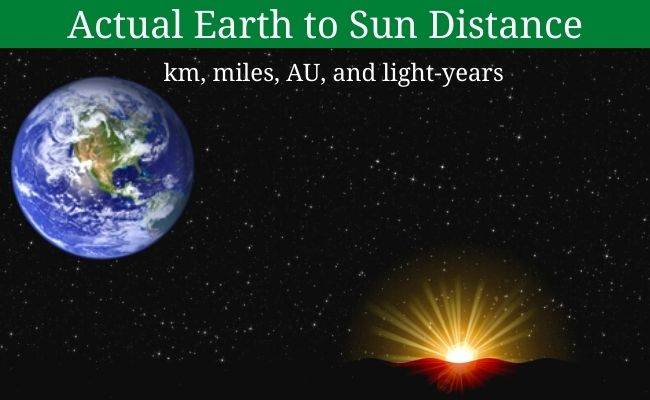# Sun Distance from Earth (km, miles, and light-years)Earth orbits the Sun in an elliptical way, it’s not a perfect circle. So, the Sun distance from Earth always varies. Though the average distance between the sun and the earth is around 149.598 million kilometers. In actuality, the Sun distance to Earth varies around 3% as the earth orbits the sun.

Usually, the earth-to-sun distance is known as one Astronomical Unit (1 AU) which is around 149.598 million kilometers (92.956 million miles). The AU is used to measure longer distances in the solar system.

## Actual Earth-to-Sun Distance

Our earth’s distance from the sun varies (you can say it, at every moment). Because the earth orbits around the sun with an average speed of around 29.78 km/sec. So, at every moment the earth either approaches near or farther from the sun.

• Earth’s closest distance from the sun is called perihelion. And farthest Sun-Earth distance is known as aphelion.

Due to the elliptical orbit path of the earth, its minimum and maximum distance vary around 3% from the average distance of 1 AU.• The closest/minimum/perihelion distance between Earth and Sun – 147.098291 million km.
• The farthest/maximum/aphelion distance between earth to the sun – 152.098233 million km.
• Average/mean earth’s distance from the sun – 149.598,023 million km.

#### Sun distance from Earth in Kilometers

• On average the sun is 149,598,023 kilometers away from the earth.
• Perihelion/Closest distance –  147,098,291 kilometers.
• Aphelion/Farthest distance – 152,098,233 kilometers.

#### Earth to Sun distance in Miles

• On average our earth is 92,955,902 miles away from the sun.
• Perihelion/Closest distance – 91,402,640 miles.
• Aphelion/Farthest distance – 94,509,460 miles.

#### Distance between Sun and Earth in AU

• On average the Sun is 1 AU away from the Earth.
• Perihelion/Closest distance – 0.9833 AU.
• Aphelion/Farthest distance – 1.0167 AU.

#### Sun-Earth distance in Light-Years

A light-year is a unit of length used to measure astronomical distances. One light-year is equal to 9.46 × 1012 kilometers. It is the distance that light can travel in a year (almost 365.25 days).

• On average the sun is 1.58137 × 10-5 light-years away from the earth.
• Perihelion/Nearest distance – 1.55495 × 10-5 light-years.
• Aphelion/Longest distance – 1.60780 × 10-5 light-years.

So, here you found the Sun’s distance from Earth in many units like kilometers, miles, AU, and light-years.

Must Check:-